# Symmetric space

(diff) ← Older revision | Latest revision (diff) | Newer revision → (diff)

A general name given to various types of spaces in differential geometry.

1) A manifold with an affine connection is called a locally symmetric affine space if the torsion tensor and the covariant derivative of the curvature tensor vanish identically.

2) A (pseudo-) Riemannian manifold is called a locally symmetric (pseudo-) Riemannian space if the covariant derivative of its curvature tensor with respect to the Levi-Civita connection vanishes identically.

3) A pseudo-Riemannian manifold (respectively, a manifold with an affine connection)is called a globally symmetric pseudo-Riemannian (affine) space if one can assign to every pointan isometry (affine transformation)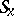ofsuch that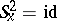andis an isolated fixed point of.

4) Letbe a connected Lie group, letbe an involutive automorphism, let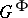be the closed subgroup of all-fixed points, letbe the component of the identity in, and letbe a closed subgroup ofsuch that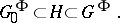Then the homogeneous space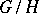is called a symmetric homogeneous space.

5) A symmetric space in the sense of Loos (a Loos symmetric space) is a manifoldendowed with a multiplication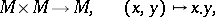satisfying the following four conditions:

a)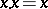;

b)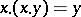;

c)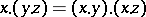;

d) every pointhas a neighbourhoodsuch thatimplies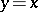for all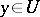.

Any globally symmetric affine (pseudo-Riemannian) space is a locally symmetric affine (pseudo-Riemannian) space and a homogeneous symmetric space. Any homogeneous symmetric space is a globally symmetric affine space and a Loos symmetric space. Every connected Loos symmetric space is a homogeneous symmetric space.

Letbe a connected Loos symmetric space, and hence a homogeneous space: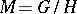. Thencan be equipped with a torsion-free invariant affine connection with the following properties:) the covariant derivative of the curvature tensor vanishes;) every geodesicis a trajectory of some one-parameter subgroupof, and parallel translation of vectors alongcoincides with their translation by means of; and) the geodesics are closed under multiplication (they are called one-dimensional subspaces). Similarly one can introduce the concept of an arbitrary subspace of, namely, a manifoldofwhich is closed under multiplication and which is a symmetric space under the induced multiplication. A closed subsetofwhich is stable under multiplication is a subspace. The analogue of the Lie algebra for a symmetric spaceis defined as follows. Letandbe the Lie algebras of the groupsand, respectively, and let(the differential at the unit), whereis the involutive automorphism defining the symmetric homogeneous space. The eigenvectors of the space endomorphismcorresponding to the eigenvalueform a subspacesuch thatis the direct sum of the subspaces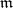and, andcan be identified with the tangent space ofat the point. If one defines a trilinear composition on the vector spaceby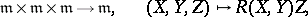whereis the curvature tensor, thenbecomes a Lie ternary system. Ifis a subspace ofpassing through the point 0, then the tangent space ofat 0 is a subsystem ofand conversely.

Ifis a Loos symmetric space, then so is the product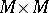. Letbe a subspace ofdefining an equivalence relation on. Thenis called a congruence. This concept is used in the construction of a theory of coverings for symmetric spaces. Two pointsare said to commute if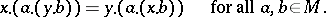The centre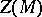ofwith respect to a pointis defined to be the set of all points ofwhich commute with 0.is a closed subspace ofwhich can be equipped with an Abelian group structure. Letbe a simply-connected symmetric space. Then the search for symmetric spaces for whichis a covering space reduces to the classification of discrete subgroups of.

In the theory of symmetric spaces, considerable attention is devoted to classification problems (see ). Letbe a locally symmetric Riemannian space. Thenis called reducible if, in some coordinate system, its fundamental quadratic form can be written as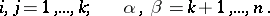Otherwise the space is called irreducible. E. Cartan has shown that the study of all irreducible locally symmetric Riemannian spaces reduces to the classification of involutive automorphisms of real compact Lie algebras, which he accomplished. At the same time he solved the local classification problem for symmetric homogeneous spaces whose fundamental groups are simple and compact. A classification of symmetric homogeneous spaces with simple non-compact fundamental groups has been obtained (see , , ).

How to Cite This Entry:
Symmetric space. Encyclopedia of Mathematics. URL: http://encyclopediaofmath.org/index.php?title=Symmetric_space&oldid=11484
This article was adapted from an original article by A.S. Fedenko (originator), which appeared in Encyclopedia of Mathematics - ISBN 1402006098. See original article